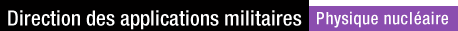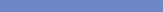} HARTREE-FOCK-BOGOLIUBOV RESULTS BASED ON THE GOGNY FORCE
Click on a nucleusHARTREE-FOCK-BOGOLIUBOV RESULTS BASED ON THE GOGNY FORCE S.Hilaire, M.GirodThis is the homepage of the "AMEDEE"  database showing the results of Hartree-Fock-Bogoliubov calculations based on the D1S  Gogny  effective nucleon-nucleon interaction. For more informations on the theoretical framework of the calculations click here . For technical details on the results shown click there. Spectroscopic properties of low energy collective levels for nearly 1700 nuclei are available here . For any other information or remark, please contact the database webmaster.

Theoretical framework

 The many body problem is solved in the framework of the mean field approximation accounting for pairing correlations, the Hartree-Fock-Bogoliubov (HFB) approximation. The self-consistent HFB equations are solved using an iterative method. They are deduced from the minimization of the total energy of the nucleus :

 δ ( < Φ | H -λZZ - λNN -μ2 Q20 | Φ >) =0

 In this expression

 • |Φ> is the HFB wave function.

 • λN and λZ are the Lagrange parameters fixing the number of neutrons N and protons Z

 • μ2 is the Lagrange parameter to fix the quadrupole moment q20 defined by

 q20 = < Φ|Q20 |Φ > with the operator Q20= (16 π / 5)½ r2 Y20

 • H is the nuclear Hamiltonian which reads

 H = ∑i Ti + ½ ∑i≠j Vij

 where Vij is the effective nucleon-nucleon interaction and Ti the kinetic energy term.

 The HFB equations are solved in an harmonic oscilator basis within the axial symetry hypothesis. The size of the basis is defiend by the number of major shells N0 used to develop the HFB wave function. This number depends on the number of nucleons contained in the nucleus. It is chosen so that the number of states in the basis is 8 time the maximum number of occupied states.

 These states also depend on the nucleus constrained deformation and are defined by the quantum numbers of the deformed harmonic oscillator ( n⊥,  m and nz ) which obey the inequation

 (2n⊥+m+1) hω⊥ +(nz+½) hωz ≤ (N0+2) hω0 with (hω0)3= (hω⊥)2 hωz

 where ω⊥ and ωz are the two parameters of the axial oscillator.

 These parameters should, in principle be determined minimizing the system total energy. This criterion is applied to determine, for each deformation, the optimal value of ω0 . However, the ratio q=ω⊥/ ωZ is not optimized but rather estimated thanks to the relation :

 q=exp [1.5 β cos(γ) / (2 β +1)] with γ=0 for β>0 and γ=π for β≤0

 based on the approximate deformation β obtained when using the Liquid drop approximation of the nucleus. In the latter approach, both the axial symetry and the parity are conserved.

 The treatment of odd-A and odd-odd nuclei is performed using the blocking procedure without breaking the time reversal symetry. This procedure consists in blocking the unpaired nucleon in a fixed orbital during the minimization procedure. Several cases are thus required to determine the blocked quasi-particle yielding the minimum energy. For odd-A nuclei, 11 configurations have been tested for each deformation, while 25 configurations are considered for odd-odd nuclei.

Technical details

 The potential energy surfaces show the nucleus HFB energy, namely

 E= < Φ | H | Φ >

 They are plotted as functions of the deformation parameter β :

 β=(5 π / 9)½ q20 / (A R02)

 where A=N+Z is the nucleus mass, R0=1.2 A1/3 its radius (expressed in fm) and q20 is the mass quadrupolar moment defined by

 q20 = < Φ|Q20 |Φ > with the operator Q20= (16 π / 5)½ r2 Y20

 When plotting only potential energy surfaces, dashed lines have been added corresponding to the approximate rotational energy correction for spins I  =  8, 16 et 24 . These are obtained adding to the nucleus binding energy the rotational energy

 Erot = [ I(I+1) ]  / 2ℑx

 given by the simplest rotational model using however the moment of inertia ℑx calculated for every deformation | β | ≥ 0.15.

 The theoretical binding energies (defined as the minimum close to β=0) as well as the experimental masses taken form the Audi-Wapstra  mass tables are also indicated.

 The chemical potentials are given by the Lagrange parameters λZ and λN for the protons and neutrons respectively.

 The quadrupolar collective masses M20 and the moments of inertia ℑx have been calculated using the Inglis-Beliaev  approximation. The determination of the zero point energies (ZPE) is also described in the same paper.

 Concerning the proton and neutron pairing energies (p/n), they are defined by

 EP(p/n) = ½ Tr ( Δ(p/n) κ(p/n) )

 where Δ(p/n) is the pairing field and κ(p/n) the pairing tensor obtained from the solution of the HFB equations.

 The β2(p/n) and β4(p/n) are deduced from the multipole moments q20 and q40 defined from the protons and neutrons distribution. More precisely,

 β4(p/n) = q40(p/n) /(A R04) with q40(p/n)= < Φ(p/n) | r4 Y40 | Φ(p/n) >

 Finally, the protons and neutrons radii are given by the square roots of the mean square radii (p/n) defined by

 < r2 >(p/n)= < Φ(p/n) | r2 | Φ(p/n) >### Select a nucleus

 Number of protons Number of neutrons